SLAS989D January   2014  – October 2017

PRODUCTION DATA.

1. Features
2. Applications
3. Description
4. Revision History
5. Pin Configuration and Functions
6. Specifications
7. Detailed Description
1. 7.1 Overview
2. 7.2 Functional Block Diagram
3. 7.3 Feature Description
1. 7.3.1 Signal Acquisition
3. 7.3.3 Clocking
4. 7.3.4 Over-Range Function
6. 7.3.6 Digital Down Converter (DDC)
1. 7.3.6.1 NCO/Mixer
2. 7.3.6.2 NCO Settings
3. 7.3.6.3 Decimation Filters
4. 7.3.6.4 DDC Output Data
5. 7.3.6.5 Decimation Settings
7. 7.3.7 Data Outputs
1. 7.3.7.1 The Digital Outputs
2. 7.3.7.2 JESD204B Interface Features and Settings
4. 7.4 Device Functional Modes
1. 7.4.1 DDC Bypass Mode
2. 7.4.2 DDC Modes
3. 7.4.3 Calibration
4. 7.4.4 Timing Calibration Mode
5. 7.4.5 Test-Pattern Modes
5. 7.5 Programming
1. 7.5.1 Using the Serial Interface
6. 7.6 Register Map
8. Application and Implementation
1. 8.1 Application Information
2. 8.2 Typical Application
2. 8.2.2 Oscilloscope
3. 8.3 Initialization Set-Up
4. 8.4 Dos and Don'ts
9. Power Supply Recommendations
10. 10Layout
11. 11Device and Documentation Support
1. 11.1 Device Support
2. 11.2 Documentation Support
3. 11.3 Community Resource
5. 11.5 Electrostatic Discharge Caution
6. 11.6 Glossary
12. 12Mechanical, Packaging, and Orderable Information

• NKE|68

6 Specifications

6.1 Absolute Maximum Ratings

The soldering process must comply with TI's Reflow Temperature Profile specifications. Refer to www.ti.com/packaging.(5)(1)(3)
MIN MAX UNIT
Supply voltage 1.2-V supply VA12, VD12 1.4 V
1.9-V supply VA19 2.2
1.2-V supply difference between VA12 and VD12 –200 200 mV
Voltage On any input pin (except VIN+ or VIN–) –0.15 V(VA19) + 0.15 V
On VIN+ or VIN– 0 2
Voltage difference |(VIN+) – (VIN–)|(2) 2 V
|(DEVCLK+) – (DEVCLK–)| 2
|(SYSREF+) – (SYSREF–)| 2
|(~SYNC+) – (~SYNC–)| 1
RF input power, PI On VIN+, VIN–, with proper input common mode maintained. FIN ≥ 3 GHz, Z(SOURCE) = 100 Ω, Input_Clamp_EN = 0 or 1 11.07 dBm
On VIN+, VIN–, with proper input common mode maintained. FIN = 1 GHz, Z(SOURCE) = 100 Ω, Input_Clamp_EN = 1 14.95
On VIN+, VIN–, with proper input common mode maintained. FIN ≤ 100 MHz, Z(SOURCE) = 100 Ω, Input_Clamp_EN = 1 20.97
Input current At any pin other than VIN+ or VIN–(4) –25 25 mA
VIN+ or VIN– –50 50 mA DC
Package(4) (sum of absolute value of all currents forced in or out, not including power supply current) 100 mA
Junction temperature, TJ Power applied. Verified by High Temperature Operation Life testing to 1000 hours. –40 150 °C
Storage temperature, Tstg –65 150 °C
Stresses beyond those listed under Absolute Maximum Ratings may cause permanent damage to the device. These are stress ratings only, and functional operation of the device at these or any other conditions beyond those indicated under Recommended Operating Conditions is not implied. Exposure to absolute-maximum-rated conditions for extended periods may affect device reliability.
The analog inputs are protected as in the following circuit. Input-voltage magnitudes beyond the Absolute Maximum Ratings may damage this device.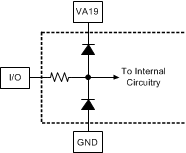If Military/Aerospace specified devices are required, please contact the Texas Instruments Sales Office/Distributors for availability and specifications.
When the input voltage at any pin (other than VIN+ or VIN–) exceeds the power supply limits (that is, less than GND or greater than VA19), the current at that pin must be limited to 25 mA. The 100-mA maximum package input current rating limits the number of pins that can safely exceed the power supplies. This limit is not placed upon the power pins or thermal pad (GND).

6.2 ESD Ratings

VALUE UNIT
V(ESD) Electrostatic discharge Human body model (HBM), per ANSI/ESDA/JEDEC JS-001, all pins(1) ±2000 V
Charged device model (CDM), per JEDEC specification JESD22-C101, all pins(2) ±500
JEDEC document JEP155 states that 500-V HBM allows safe manufacturing with a standard ESD control process.
JEDEC document JEP157 states that 250-V CDM allows safe manufacturing with a standard ESD control process.

6.3 Recommended Operating Conditions

All voltages are measured with respect to GND = 0 V, unless otherwise specified.
MIN MAX UNIT
VDD Supply voltage 1.2-V supply: VA12, VD12 1.14 1.26 V
1.9-V supply: VA19 1.8 2
Supply sequence (power-up and power-down) 1.9 supply ≥ 1.2 supply V
VCMI Analog input common mode voltage V(VCMO) – 0.15 V(VCMO) + 0.15 V
VIN+, VIN– voltage (maintaining common mode) 0 V(VA19) V
DEVCLK±, SYSREF±, ~SYNC± pin voltage range 0 V(VA19) V
VID(CLK) Differential DEVCLK±, SYSREF±, ~SYNC± amplitude 0.4 2 VPP
VCM(CLK) SYSREF±, ~SYNC± Common Mode 0.64 1.1 V
TA Ambient temperature –40 85 °C
TJ Junction temperature 135 °C

6.4 Thermal Information

NKE (VQFN)
68 PINS
RθJA Thermal resistance, junction-to-ambient 19.8 °C/W
RθJCbot Thermal resistance, junction-to-case (bottom) 2.7 °C/W
ψJB Characterization parameter, junction-to-board 9.1 °C/W

6.5 Electrical Characteristics

Unless otherwise noted, these specifications apply for V(VA12) = V(VD12) = 1.2 V, V(VA19) = 1.9 V, VIN full scale range at default setting (725 mVPP), VIN = –1 dBFS, differential AC-coupled sinewave input clock, ƒ(DEVCLK) = 4 GHz at 0.5 VPP with 50% duty cycle, R(RBIAS) = 3.3 kΩ ±0.1%, after a foreground (FG) mode calibration with timing calibration enabled. Typical values are at TA = 25°C.(1)(3)
PARAMETER TEST CONDITIONS MIN TYP MAX UNIT
DYNAMIC PERFORMANCE CHARACTERISTICS
RES ADC core resolution Resolution with no missing codes 12 Bits
INL Integral non-linearity TA = 25°C ±2 LSB
TA = TMIN to TMAX ±3
DNL Differential non-linearity TA = 25°C ±0.25 LSB
TA = TMIN to TMAX ±0.3
Peak NPR Peak noise power ratio 500-kHz tone spacing from 1 MHz to ƒS / 2−1 MHz, DDC bypass mode
25-MHz wide notch at 320 MHz
46 dB
IMD3 Third-order intermodulation distortion F1 = 2110 MHz at −13 dBFS
F2 = 2170 MHz at −13 dBFS
–64 dBc
DDC BYPASS MODE
SNR1 Signal-to-noise ratio, integrated across entire Nyquist bandwidth
Input frequency-dependent interleaving spurs included
FIN = 350 MHz, –1 dBFS, 12-bit DDC bypass mode 55 dBFS
FIN = 600 MHz, –1 dBFS, 12-bit DDC bypass mode TA = 25°C 54.8
TA = TMIN to TMAX 52.5
TA = 25°C, calibration = BG 53.9
TA = TMIN to TMAX, calibration = BG 49.4
FIN = 1500 MHz, –1 dBFS, 12-bit DDC bypass mode 51.2
FIN = 2400 MHz, –1 dBFS, 12-bit DDC bypass mode 48.7
SNR2 Signal-to-noise ratio, integrated across entire Nyquist bandwidth
Input frequency-dependent interleaving spurs excluded
FIN = 600 MHz, –1 dBFS, 12-bit DDC bypass mode TA = 25°C(4) 55 dBFS
TA = TMIN to TMAX(4) 53
TA = 25°C, calibration = BG(4) 55
TA = TMIN to TMAX, calibration = BG(4) 53
SINAD1 Signal-to-noise and distortion ratio, integrated across entire Nyquist bandwidth
Input frequency-dependent interleaving spurs included
FIN = 350 MHz, –1 dBFS, 12-bit DDC bypass mode 54.8 dBFS
FIN = 600 MHz, –1 dBFS, 12-bit DDC bypass mode TA = 25°C 54.7
TA = TMIN to TMAX 52.3
TA = 25°C, calibration = BG 53.8
TA = TMIN to TMAX, calibration = BG 49.2
FIN = 1500 MHz, –1 dBFS, 12-bit DDC bypass mode 51.1
FIN = 2400 MHz, –1 dBFS, 12-bit DDC bypass mode 48.7
SINAD2 Signal-to-noise and distortion ratio, integrated across DDC output bandwidth
Input frequency-dependent interleaving spurs excluded
FIN = 600 MHz, –1 dBFS, 12-bit DDC bypass mode TA = 25°C(4) 54.9 dBFS
TA = TMIN to TMAX(4) 52.7
TA = 25°C, calibration = BG(4) 54.9
TA = TMIN to TMAX, calibration = BG(4) 52.7
ENOB1 Effective number of bits, integrated across entire Nyquist bandwidth
Input frequency-dependent interleaving spurs included
FIN = 350 MHz, –1 dBFS, 12-bit DDC bypass mode 8.8 Bits
FIN = 600 MHz, –1 dBFS, 12-bit DDC bypass mode TA = 25°C 8.8
TA = TMIN to TMAX 8.4
TA = 25°C, calibration = BG 8.7
TA = TMIN to TMAX, calibration = BG 7.9
FIN = 1500 MHz, –1 dBFS, 12-bit DDC bypass mode 8.2
FIN = 2400 MHz, –1 dBFS, 12-bit DDC bypass mode 7.8
ENOB2 Effective number of bits, integrated across entire Nyquist bandwidth
Input frequency-dependent interleaving spurs excluded
FIN = 600 MHz, –1 dBFS, 12-bit DDC bypass mode TA = 25°C(4) 8.8 Bits
TA = TMIN to TMAX(4) 8.5
TA = 25°C, calibration = BG(4) 8.8
TA = TMIN to TMAX, calibration = BG(4) 8.5
SFDR1 Spurious-free dynamic range
Input frequency-dependent interleaving spurs included
FIN = 350 MHz, –1 dBFS, 12-bit DDC bypass mode 67.4 dBFS
FIN = 600 MHz, –1 dBFS, 12-bit DDC bypass mode TA = 25°C 70.7
TA = TMIN to TMAX 60
TA = 25°C, calibration = BG 63.4
TA = TMIN to TMAX, calibration = BG 51.8
FIN = 1500 MHz, –1 dBFS, 12-bit DDC bypass mode 59.8
FIN = 2400 MHz, –1 dBFS, 12-bit DDC bypass mode 57.2
SFDR2 Spurious-free dynamic range
Input frequency-dependent interleaving spurs excluded
FIN = 600 MHz, –1 dBFS, 12-bit DDC bypass mode TA = 25°C(4) 73 dBFS
TA = TMIN to TMAX(4) 61.6
TA = 25°C, calibration = BG(4) 74
TA = TMIN to TMAX, calibration = BG (4)mode 62.8
ƒS/2 Interleaving offset spur at ½ sampling rate FIN = 350 MHz, –1 dBFS, 12-bit DDC bypass mode –75 dBFS
FIN = 600 MHz, –1 dBFS, 12-bit DDC bypass mode TA = 25°C –76
TA = TMIN to TMAX –60
TA = 25°C, calibration = BG –68
TA = TMIN to TMAX, calibration = BG –55
FIN = 1500 MHz, –1 dBFS, 12-bit DDC bypass mode –75
FIN = 2400 MHz, –1 dBFS, 12-bit DDC bypass mode –75
ƒS/4 Interleaving offset spur at ¼ sampling rate FIN = 350 MHz, –1 dBFS, 12-bit DDC bypass mode –68 dBFS
FIN = 600 MHz, –1 dBFS, 12-bit DDC bypass mode TA = 25°C –70
TA = TMIN to TMAX –55
TA = 25°C, calibration = BG –61
TA = TMIN to TMAX, calibration = BG –47.4
FIN = 1500 MHz, –1 dBFS, 12-bit DDC bypass mode –68
FIN = 2400 MHz, –1 dBFS, 12-bit DDC bypass mode –68
ƒS/2 – FIN Interleaving offset spur at ½ sampling rate – input frequency FIN = 600 MHz, –1 dBFS, 12-bit DDC bypass mode TA = 25°C –77 dBFS
TA = TMIN to TMAX –61.7
TA = 25°C, calibration = BG –70
TA = TMIN to TMAX, calibration = BG –51.9
ƒS/4 + FIN Interleaving offset spur at ¼ sampling rate + input frequency FIN = 600 MHz, –1 dBFS, 12-bit DDC bypass mode TA = 25°C –74 dBFS
TA = TMIN to TMAX –60
TA = 25°C, calibration = BG –66
TA = TMIN to TMAX, calibration = BG –52
ƒS/4 – FIN Interleaving offset spur at ¼ sampling rate – input frequency FIN = 600 MHz, –1 dBFS, 12-bit DDC bypass mode TA = 25°C –76 dBFS
TA = TMIN to TMAX –60.4
TA = 25°C, calibration = BG –67
TA = TMIN to TMAX, calibration = BG –53.3
THD Total harmonic distortion FIN = 350 MHz, –1 dBFS, 12-bit DDC bypass mode –72 dBFS
FIN = 600 MHz, –1 dBFS, 12-bit DDC bypass mode TA = 25°C –70
TA = TMIN to TMAX –60
TA = 25°C, calibration = BG –72
TA = TMIN to TMAX, calibration = BG –60
FIN = 1500 MHz, –1 dBFS, 12-bit DDC bypass mode –68
FIN = 2400 MHz, –1 dBFS, 12-bit DDC bypass mode –74
HD2 Second harmonic distortion FIN = 350 MHz, –1 dBFS, 12-bit DDC bypass mode –85 dBFS
FIN = 600 MHz, –1 dBFS, 12-bit DDC bypass mode TA = 25°C –80
TA = TMIN to TMAX –62
TA = 25°C, calibration = BG –80
TA = TMIN to TMAX, calibration = BG –62.5
FIN = 1500 MHz, –1 dBFS, 12-bit DDC bypass mode –71
FIN = 2400 MHz, –1 dBFS, 12-bit DDC bypass mode –79
HD3 Third harmonic distortion FIN = 350 MHz, –1 dBFS, 12-bit DDC bypass mode –73 dBFS
FIN = 600 MHz, –1 dBFS, 12-bit DDC bypass mode TA = 25°C –75
TA = TMIN to TMAX –61
TA = 25°C, calibration = BG –80
TA = TMIN to TMAX, calibration = BG –61.7
FIN = 1500 MHz, –1 dBFS, 12-bit DDC bypass mode –74
FIN = 2400 MHz, –1 dBFS, 12-bit DDC bypass mode –76
NSD Noise spectral density, average NSD across Nyquist bandwidth 12-bit DDC bypass mode 50-Ω AC-coupled terminated input –149 dBFS/Hz
–150.8 dBm/Hz
FIN = 600 MHz, –1 dBFS –147.8 dBFS/Hz
–149.6 dBm/Hz
DECIMATE-BY-8 MODE
SNR1 Signal-to-noise ratio, integrated across DDC output bandwidth
Interleaving spurs included
FIN = 600 MHz, –1 dBFS, decimate-by-8 mode 63 dBFS
Calibration = BG 61.6
FIN = 2400 MHz, –1 dBFS, decimate-by-8 mode 54.6
SNR2 Signal-to-noise ratio, integrated across DDC output bandwidth
Interleaving spurs excluded
FIN = 600 MHz, –1 dBFS, decimate-by-8 mode(4) 63.3 dBFS
Calibration = BG 63.3
SINAD1 Signal-to-noise and distortion ratio, integrated across DDC output bandwidth
Interleaving spurs included
FIN = 600 MHz, –1 dBFS, Decimate-by-8 mode 63 dBFS
Calibration = BG 61.6
FIN = 2400 MHz, –1 dBFS, decimate-by-8 mode 54.6
SINAD2 Signal-to-noise and distortion ratio, integrated across DDC output bandwidth
Interleaving spurs excluded
FIN = 600 MHz, –1 dBFS, decimate-by-8 mode(4) 63.3 dBFS
Calibration = BG 63.3
ENOB1 Effective number of bits, integrated across DDC output bandwidth
Interleaving spurs included
FIN = 600 MHz, –1 dBFS, decimate-by-8 mode 10.2 Bits
Calibration = BG 10.0
FIN = 2400 MHz, –1 dBFS, decimate-by-8 mode 8.8
ENOB2 Effective number of bits, integrated across DDC output bandwidth
Interleaving spurs excluded
FIN = 600 MHz, –1 dBFS, decimate-by-8 mode(5) 10.2 Bits
Calibration = BG 10.2
SFDR1 Spurious-free dynamic range
Interleaving Spurs Included
FIN = 600 MHz, –1 dBFS, decimate-by-8 mode 74.9 dBFS
Calibration = BG 68.3
SFDR2 Spurious-free dynamic range
Interleaving spurs excluded
FIN = 600 MHz, –1 dBFS, decimate-by-8 mode(5) 77.8 dBFS
Calibration = BG 77.8
ƒS/2 Interleaving offset spur at ½ sampling rate(5) FIN = 600 MHz, –1 dBFS, decimate-by-8 mode –73 dBFS
Calibration = BG –72
ƒS/4 Interleaving offset spur at ¼ sampling rate(5) FIN = 600 MHz, –1 dBFS, decimate-by-8 mode –70 dBFS
Calibration = BG –66
ƒS/2 – FIN Interleaving spur at ½ sampling rate – input frequency(5) FIN = 600 MHz, –1 dBFS, decimate-by-8 mode –76 dBFS
Calibration = BG –67
ƒS/4 + FIN Interleaving spur at ¼ sampling rate + input frequency(5) FIN = 600 MHz, –1 dBFS, decimate-by-8 mode –72 dBFS
Calibration = BG –64
ƒS/4 – FIN Interleaving spur at ¼ sampling rate – input frequency(5) FIN = 600 MHz, –1 dBFS, decimate-by-8 mode –74 dBFS
Calibration = BG –67
THD Total harmonic distortion(6) FIN = 600 MHz, –1 dBFS, decimate-by-8 mode –70 dBFS
Calibration = BG –72
FIN = 2400 MHz, –1 dBFS, decimate-by-8 mode –71
HD2 Second harmonic distortion(6) FIN = 600 MHz, –1 dBFS, decimate-by-8 mode –80 dBFS
Calibration = BG –79
FIN = 2400 MHz, –1 dBFS, decimate-by-8 mode –78
HD3 Third harmonic distortion(6) FIN = 600 MHz, –1 dBFS, decimate-by-8 mode –74 dBFS
Calibration = BG –80
FIN = 2400 MHz, –1 dBFS, decimate-by-8 mode –-77
DDC CHARACTERISTICS
Alias protection(2) 80 dB
Alias protected bandwidth(2) 80 % of output BW
SFDR-DDC Spurious-free dynamic range of digital down-converter(2) 100 dB
Implementation loss(2) 0.5 dB
VID(VIN) Full-scale analog-differential input range Minimum FSR setting(6) 500 mVPP
Default FSR setting, TA = TMIN to TMAX 650 725 800
Maximum FSR setting(6) 950
Each input pin to ground 1.5 pF
RID(VIN) Differential input resistance 80 95 110 Ω
FPBW Full power bandwidth –3 dB — calibration = BG 2.8 GHz
–3 dB — calibration = FG 3.2
Gain flatness DC to 2 GHz 1.2 dB
2 GHz to 4 GHz 3.8
DC to 2 GHz — calibration = BG 1.5
2 GHz to 4 GHz — calibration = BG 4.5
ANALOG OUTPUT CHARACTERISTICS (VCMO, VBG)
V(VCMO) Common-mode output voltage I(VCMO) = ±100 µA, TA = 25°C 1.225 V
I(VCMO) = ±100 µA, TA = TMIN to TMAX 1.185 1.265
TCVO(VCMO) Common-mode output-voltage temperature coefficient TA = TMIN to TMAX -21 ppm/°C
VO(BG) Bandgap reference output voltage I(BG) = ±100 µA, TA = 25°C 1.248 V
I(BG) = ±100 µA, TA = TMIN to TMAX 1.195 1.3
TCVref(BG) Bandgap reference voltage temperature coefficient TA = TMIN to TMAX,
I(BG) = ±100 µA
0 ppm/°C
TEMPERATURE DIODE CHARACTERISTICS
V(TDIODE) Temperature diode voltage slope Offset voltage (approx. 0.77 V) varies with process and must be measured for each part. Offset measurement should be done with PowerDown=1 to minimize device self-heating. 100-µA forward current
Device active
–1.6 mV/°C
100-µA forward current
Device in power-down
–1.6 mV/°C
CLOCK INPUT CHARACTERISTICS (DEVCLK±, SYSREF±, SYNC~/TMST±)
VID(CLK) Differential clock input level Sine wave clock, TA = TMIN to TMAX 0.4 0.6 2 VPP
Square wave clock, TA = TMIN to TMAX 0.4 0.6 2 VPP
II(CLK) Input current VI = 0 or VI = VA ±1 µA
CI(CLK) Input capacitance(2) Differential 0.02 pF
Each input to ground 1 pF
RID(CLK) Differential input resistance TA = 25°C 95 Ω
TA = TMIN to TMAX 80 110 Ω
CML OUTPUT CHARACTERISTICS (DS0–DS7±)
VOD Differential output voltage Assumes ideal 100-Ω load
Measured differentially
Default pre-emphasis setting
280 305 330 mV peak
VO(ofs) Output offset voltage 0.6 V
IOS Output short-circuit current Output+ and output– shorted together ±6 mA
Output+ or output– shorted to 0 V 12
ZOD Differential output impedance 100 Ω
LVCMOS INPUT CHARACTERISTICS (SDI, SCLK, SCS, SYNC~)
VIH Logic high input voltage See (6) 0.83 V
VIL Logic low input voltage See (6) 0.4 V
CI Input capacitance(2)(7) Each input to ground 1 pF
LVCMOS OUTPUT CHARACTERISTICS (SDO, OR_T0, OR_T1)
VOH CMOS H level output IOH = –400 µA(6) 1.65 1.9 V
VOL CMOS L level output IOH = 400 µA(6) 0.01 0.15 V
POWER SUPPLY CHARACTERISTICS
I(VA19) Analog 1.9-V supply current PD = 0, calibration = FG, bypass DDC 461 500 mA
PD = 0, calibration = BG, bypass DDC 560 600
PD = 0, calibration = BG, decimate by 8, DDR = 0, P54 = 1 560 607
I(VA12) Analog 1.2-V supply current PD = 0, calibration = FG, bypass DDC 320 385 mA
PD = 0, calibration = BG, bypass DDC 364 420
PD = 0, calibration = BG, decimate by 8, DDR = 0, P54 = 1 377 428
I(VD12) Digital 1.2-V supply current PD = 0, calibration = FG, bypass DDC 445 710 mA
PD = 0, calibration = BG, bypass DDC 458 732
PD = 0, calibration = BG, decimate by 8, DDR = 0, P54 = 1 541 826
PC Power consumption PD = 0, calibration = FG, bypass DDC 1.8 2.26 W
PD = 0, calibration = BG, bypass DDC 2.05 2.52
PD = 0, calibration = BG, decimate by 8, DDR = 0, P54 = 1 2.17 2.66
PD = 1 < 50 mW
To ensure accuracy, the VA19, VA12, and VD12 pins are required to be well bypassed. Each supply pin must be decoupled with one or more bypass capacitors.
This parameter is specified by design and is not tested in production.
Interleave related fixed frequency spurs at ƒS / 4 and ƒS / 2 are excluded from all SNR, SINAD, ENOB and SFDR specifications. The magnitude of these spurs is provided separately.
Interleave related spurs at ƒS / 2 – FIN, ƒS / 4 + FIN and ƒS / 4 – FIN are excluded from these performance calculations. The magnitude of these spurs is provided separately.
Magnitude of reported tones in output spectrum of ADC core. This tone will only be present in the DDC output for specific Decimation and NCO settings. Careful frequency planning can be used to intentionally place unwanted tones outside the DDC output spectrum.
This parameter is specified by design, characterization, or both and is not tested in production.
The digital control pin capacitances are die capacitances only and is in addition to package and bond-wire capacitance of approximately 0.4 pF.

6.6 Timing Requirements

MIN NOM MAX UNIT
DEVICE (SAMPLING) CLOCK
ƒ(DEVCLK) Input DEVCLK frequency Sampling rate is equal to clock input 1 4 GHz
td(A) Sampling (aperture) delay Input CLK transition to sampling instant 0.64 ns
t(AJ) Aperture jitter 0.1 ps RMS
t(LAT) ADC core latency(2) Decimation = 1, DDR = 1, P54 = 0 64 t(DEVCLK)
t(LAT_DDC) ADC core and DDC latency(2) Decimation = 4, DDR = 1, P54 = 0 292 t(DEVCLK)
Decimation = 4, DDR = 1, P54 = 1 284
Decimation = 8, DDR = 0, P54 = 0 384
Decimation = 8, DDR = 0, P54 = 1 368
Decimation = 8, DDR = 1, P54 = 0 392
Decimation = 8, DDR = 1, P54 = 1 368
Decimation = 10, DDR = 0, P54 = 0 386
Decimation = 10, DDR = 1, P54 = 0 386
Decimation = 16, DDR = 0, P54 = 0 608
Decimation = 16, DDR = 0, P54 = 1 560
Decimation = 16, DDR = 1, P54 = 0 608
Decimation = 16, DDR = 1, P54 = 1 560
Decimation = 20, DDR = 0, P54 = 0 568
Decimation = 20, DDR = 1, P54 = 0 568
Decimation = 32, DDR = 0, P54 = 0 1044
Decimation = 32, DDR = 0, P54 = 1 948
Decimation = 32, DDR = 1, P54 = 0 1044
JESD204B INTERFACE LINK TIMING CHARACTERISTICS (REFER TO Figure 1)
td(LMFC) SYSREF to LMFC delay
Functional delay between SYSREF assertion latched and LMFC frame boundary(2)
All decimation modes 40 t(DEVCLK)
td(TX) LMFC to frame boundary delay - DDC bypass mode
Functional delay from LMFC frame boundary to beginning of next multi-frame in transmitted data.(3)
Decimation = 1, DDR = 1, P54 = 0 52.7 t(DEVCLK)
td(TX) LMFC to frame boundary delay - decimation modes
Functional delay from LMFC frame boundary to beginning of next multi-frame in transmitted data(3)
Decimation = 4, DDR = 1, P54 = 0 52.7 t(DEVCLK)
Decimation = 4, DDR = 1, P54 = 1 43.9
Decimation = 8, DDR = 0, P54 = 0 60.7
Decimation = 8, DDR = 0, P54 = 1 51.5
Decimation = 8, DDR = 1, P54 = 0 52.7
Decimation = 8, DDR = 1, P54 = 1 43.9
Decimation = 10, DDR = 0, P54 = 0 60.7
Decimation = 10, DDR = 1, P54 = 0 52.7
Decimation = 16, DDR = 0, P54 = 0 60.7
Decimation = 16, DDR = 0, P54 = 1 51.5
Decimation = 16, DDR = 1, P54 = 0 52.7
Decimation = 16, DDR = 1, P54 = 1 43.9
Decimation = 20, DDR = 0, P54 = 0 60.7
Decimation = 20, DDR = 1, P54 = 0 52.7
Decimation = 32, DDR = 0, P54 = 0 60.7
Decimation = 32, DDR = 0, P54 = 1 51.5
Decimation = 32, DDR = 1, P54 = 0 52.7
tsu(SYNC~-F) SYNC~ to LMFC setup time(1)
Required SYNC~ setup time relative to the internal LMFC boundary.
40 t(DEVCLK)
th(SYNC~-F) SYNC~ to LMFC hold time(1)
Required SYNC~ hold time relative to the internal LMFC boundary.
–8
t(SYNC~) SYNC~ assertion time
Required SYNC~ assertion time before deassertion to initiate a link resynchronization.
4 Frame clock cycles
td(LMFC) Delay from SYSREF sampled high by DEVCLK to internal LMFC boundary 40 t(DEVCLK)
t(ILA) Duration of initial lane alignment sequence 4 Multi-frame clock cycles
SYSREF
tsu(SYS) Setup time SYSREF relative to DEVCLK rising edge(6) 40 ps
th(SYS) Hold time SYSREF relative to DEVCLK rising edge(6) 40 ps
t(PH_SYS) SYSREF assertion duration after rising edge event. 8 t(DEVCLK)
t(PL_SYS) SYSREF deassertion duration after falling edge event. 8 t(DEVCLK)
t(SYS) Period SYSREF± DDR = 0, P54 = 0 K × F × 10 t(DEVCLK)
DDR = 0, P54 = 1 K × F × 8
DDR = 1, P54 = 0 K × F × 5
DDR = 1, P54 = 1 K × F × 4
SERIAL INTERFACE (REFER TO Figure 2)
ƒ(SCK) Serial clock frequency(2) 20 MHz
t(PH) Serial clock high time 20 ns
t(PL) Serial clock low time 20 ns
tsu Serial-data to serial-clock rising setup time(2) 10 ns
th Serial-data to serial clock rising hold time(2) 10 ns
t(CSS) SCS-to-serial clock rising setup time 10 ns
t(CSH) SCS-to-serial clock falling hold time 10 ns
t(IAG) Inter-access gap 10 ns
This parameter must be met to achieve deterministic alignment of the data frame and NCO phase to other similar devices. If this parameter is not met the device will still function correctly but will not be aligned to other devices.
Unless otherwise specified, delays quoted are exact un-rounded functional delays (assuming zero propagation delay).
The values given are functional delays only. Additional propagation delay of 0 to 3 clock cycles will be present.

6.7 Internal Characteristics

PARAMETER TEST CONDITIONS MIN NOM MAX UNIT
DEVICE (SAMPLING) CLOCK
td(A) Sampling (aperture) delay Input CLK transition to sampling instant 0.64 ns
t(AJ) Aperture jitter 0.1 ps RMS
t(LAT) ADC core latency. See (2) Decimation = 1, DDR = 1, P54 = 0 64 t(DEVCLK)
CALIBRATION TIMING CHARACTERISTICS (REFER TO THE CALIBRATION SECTION)
t(CAL) Calibration cycle time Calibration = FG, T_AUTO=1 227 × 106 t(DEVCLK)
Calibration = FG, T_AUTO=0 102 × 106
JESD204B INTERFACE LINK TIMING CHARACTERISTICS (REFER TO Figure 1)
td(LMFC) SYSREF to LMFC delay
Functional delay between SYSREF assertion latched and LMFC frame boundary(2)
All decimation modes 40 t(DEVCLK)
td(TX) LMFC to Frame Boundary delay - DDC Bypass Mode
Functional delay from LMFC frame boundary to beginning of next multi-frame in transmitted data(3)
Decimation = 1, DDR = 1, P54 = 0 52.7 t(DEVCLK)
td(TX) LMFC to frame boundary delay - decimation modes
Functional delay from LMFC frame boundary to beginning of next multi-frame in transmitted data(3)
Decimation = 4, DDR = 1, P54 = 0 52.7 t(DEVCLK)
Decimation = 4, DDR = 1, P54 = 1 43.9
Decimation = 8, DDR = 0, P54 = 0 60.7
Decimation = 8, DDR = 0, P54 = 1 51.5
Decimation = 8, DDR = 1, P54 = 0 52.7
Decimation = 8, DDR = 1, P54 = 1 43.9
Decimation = 10, DDR = 0, P54 = 0 60.7
Decimation = 10, DDR = 1, P54 = 0 52.7
Decimation = 16, DDR = 0, P54 = 0 60.7
Decimation = 16, DDR = 0, P54 = 1 51.5
Decimation = 16, DDR = 1, P54 = 0 52.7
Decimation = 16, DDR = 1, P54 = 1 43.9
Decimation = 20, DDR = 0, P54 = 0 60.7
Decimation = 20, DDR = 1, P54 = 0 52.7
Decimation = 32, DDR = 0, P54 = 0 60.7
Decimation = 32, DDR = 0, P54 = 1 51.5
Decimation = 32, DDR = 1, P54 = 0 52.7
td(LMFC) Delay from SYSREF sampled high by DEVCLK to internal LMFC boundary 40 t(DEVCLK)
t(ILA) Duration of initial lane alignment sequence 4 Multi-frame clock cycles

6.8 Switching Characteristics

Unless otherwise noted, these specifications apply for V(VA12) = V(VD12) = 1.2 V, V(VA19) = 1.9 V, VIN FSR (AC coupled) = Default setting, differential AC-coupled sinewave input clock, ƒ(DEVCLK) = 4 GHz at 0.5 VPP with 50% duty cycle, R(RBIAS) = 3.3 kΩ ±0.1%, after a foreground mode calibration with timing calibration enabled. Typical values are at TA = 25°C.
PARAMETER TEST CONDITIONS MIN TYP MAX UNIT
SERIAL DATA OUTPUTS
Serialized output bit rate 1 10 Gbps
Serialized output bit rate DDR = 0, P54 = 0 ƒS
DDR = 0, P54 = 1 1.25 × ƒS
DDR = 1, P54 = 0 2 × ƒS
DDR = 1, P54 = 1 2.5 × ƒS
tTLH LH transition time — differential 10% to 90%, 8 Gbps 35 ps
tTHL HL transition time — differential 10% to 90%, 8 Gbps 35 ps
UI Unit interval 8 Gbps serial rate 125 ps
DDJ Data dependent jitter 8 Gbps serial rate 11.3 ps
RJ Random Jitter 8 Gbps serial rate 1.4 ps
SERIAL INTERFACE
t(OZD) SDO tri-state to driven See Figure 2 5 ns
t(ODZ) SDO driven to tri-state 2.5 5 ns
t(OD) SDO output delay 20 ns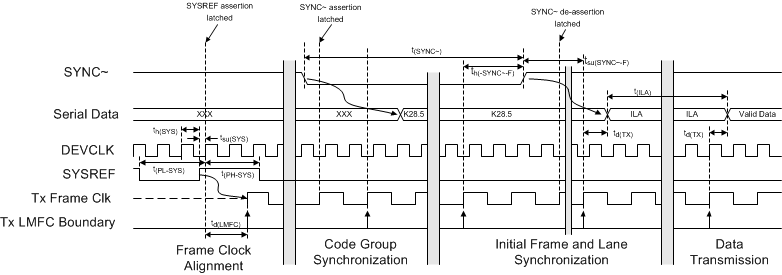Figure 1. JESD204 Synchronization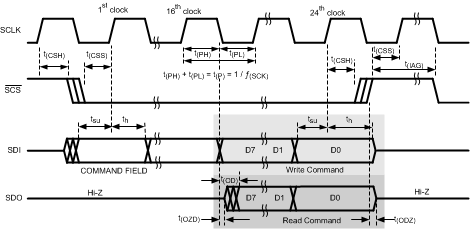Figure 2. Serial Interface Timing

6.9 Typical Characteristics

Unless otherwise noted, these specifications apply for V(VA12) = V(VD12) = 1.2 V, V(VA19) = 1.9 V, VIN FSR (AC coupled) = Default setting, differential AC-coupled sinewave input clock, ƒ(DEVCLK) = 4 GHz at 0.5 VPP with 50% duty cycle, R(RBIAS) = 3.3 kΩ ±0.1%, after a Foreground mode calibration with Timing Calibration enabled. TA = 25°C. VI = –1 dBFS.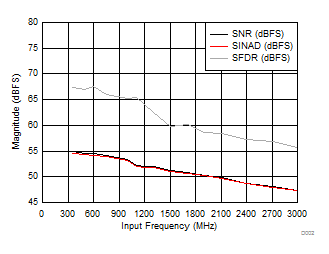DDC bypass mode Sampling rate = 4000 MSPS
Figure 3. SNR, SINAD, SFDR vs Input FrequencyDDC bypass mode FIN = 608 MHz
Figure 5. SNR, SINAD, SFDR vs Decimation Setting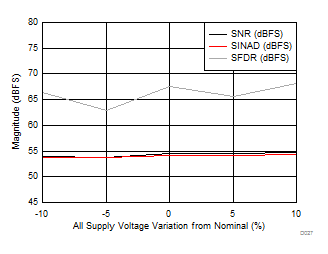DDC bypass mode FIN = 608 MHz
Figure 7. SNR, SINAD, SFDR vs Supply Voltage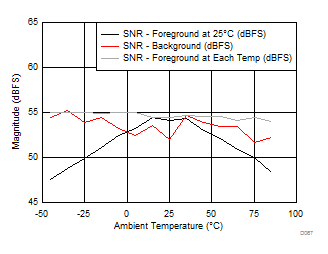DDC bypass mode FIN = 351 MHz
Figure 9. SNR vs Temperature and Calibration Mode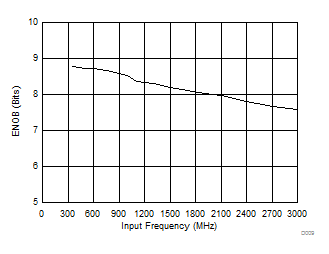DDC bypass mode Sampling rate = 4000 MSPS
Figure 11. ENOB vs Input Frequency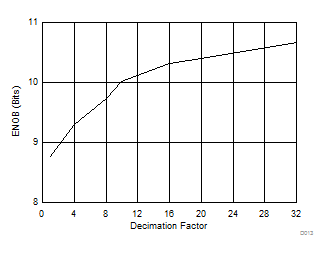FIN = 608 MHz
Figure 13. ENOB vs Decimation Setting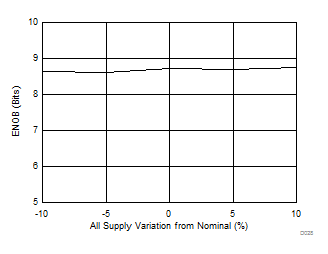DDC bypass mode FIN = 608 MHz
Figure 15. ENOB vs Supply Voltage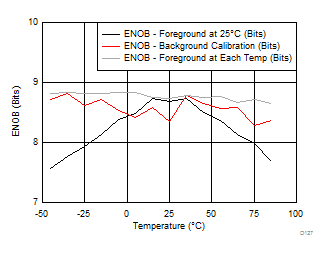DDC bypass mode FIN = 351 MHz
Figure 17. ENOB vs Temperature and Calibration Mode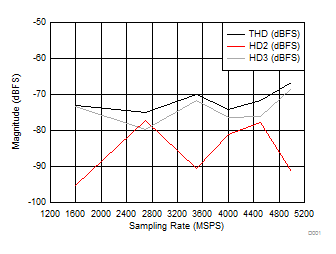DDC bypass mode FIN = 608 MHz
Figure 19. THD, HD2, HD3 vs Sampling Rate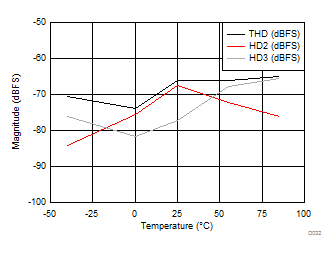DDC bypass mode FIN = 608 MHz
Figure 21. THD, H2, H3 vs Temperature - FIN = 607.77MHzDDC bypass mode FIN = 351 MHz
Figure 23. H3 vs Temperature and Calibration Mode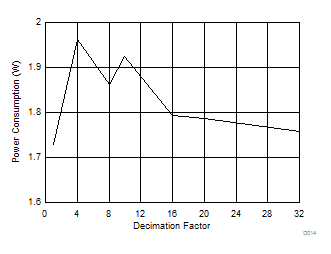FIN = 608 MHz
Figure 25. Power Consumption vs Decimation Setting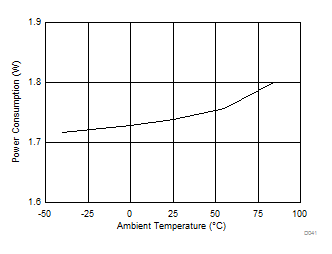DDC bypass mode
Figure 27. Power Consumption vs TemperatureForeground calibration mode
Figure 29. Supply Current vs Decimation Setting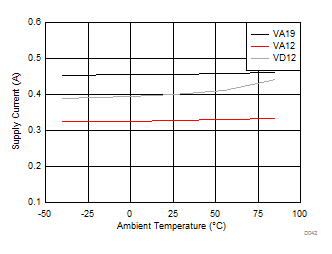DDC bypass mode Foreground calibration mode
Figure 31. Supply Current vs Temperature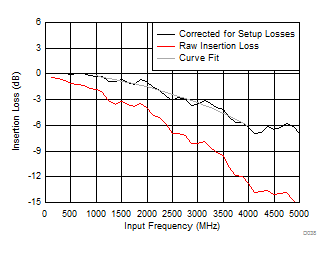Background calibration mode
Figure 33. Insertion Loss vs Input Frequency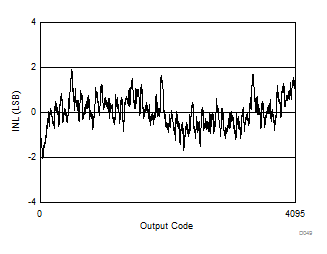Figure 35. INL versus Code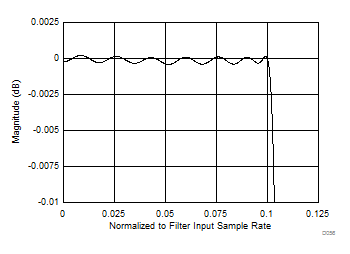Figure 37. Decimate by 4 - Passband Response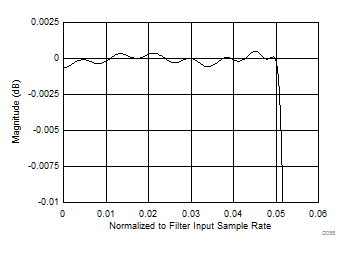Figure 39. Decimate by 8 - Passband Response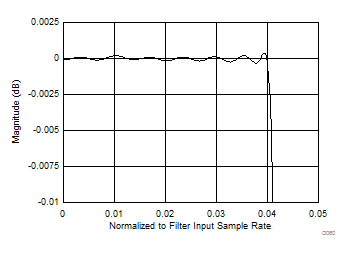Figure 41. Decimate by 10 - Passband ResponseFigure 43. Decimate by 16 - Passband Response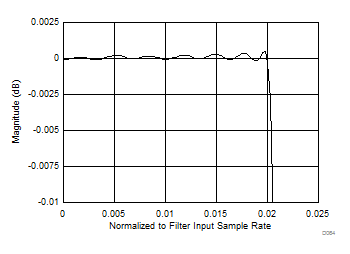Figure 45. Decimate by 20 - Passband Response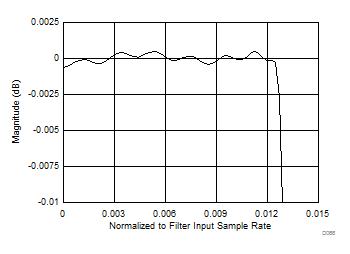Figure 47. Decimate by 32 - Passband Response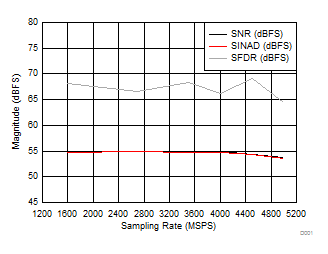DDC bypass mode FIN = 608 MHz
Figure 4. SNR, SINAD, SFDR vs Sampling rate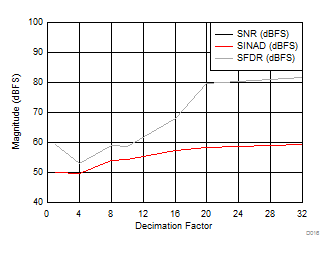DDC bypass mode FIN = 2483 MHz
Figure 6. SNR, SINAD, SFDR vs Decimation Setting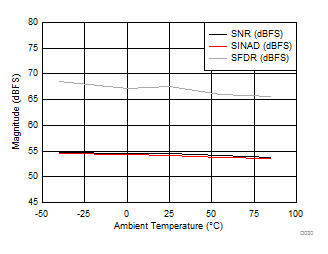DDC bypass mode FIN = 608 MHz
Figure 8. SNR, SINAD, SFDR vs Temperature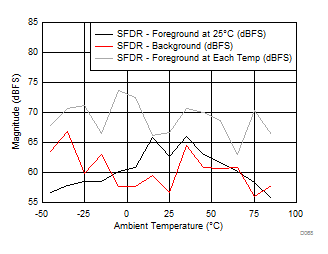DDC bypass mode Input frequency = 351 MHz
Figure 10. SFDR vs Temperature and Calibration Mode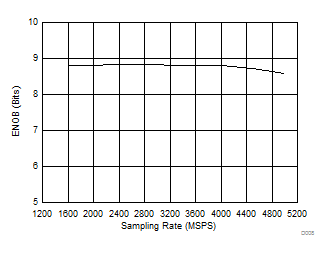DDC bypass mode FIN = 608 MHz
Figure 12. ENOB vs Sampling Rate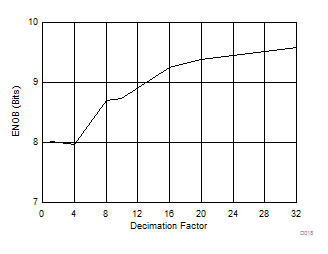FIN = 2483 MHz
Figure 14. ENOB vs Decimation Setting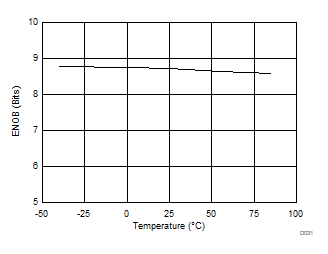DDC bypass mode FIN = 608 MHz
Figure 16. ENOB vs Temperature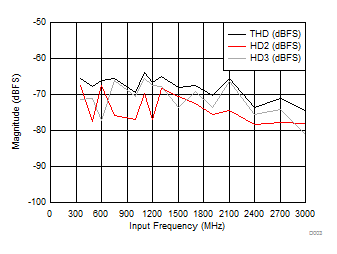DDC bypass mode Sampling rate = 4000 MSPS
Figure 18. THD, HD2, HD3 vs Input Frequency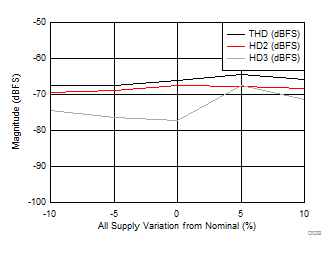DDC bypass mode FIN = 608 MHz
Figure 20. THD, H2, H3 vs Supply Voltage - FIN = 607.77MHzDDC bypass mode FIN = 351 MHz
Figure 22. H2 vs Temperature and Calibration Mode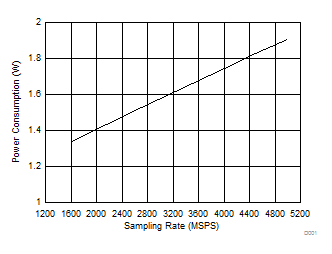DDC bypass mode FIN = 600 MHz
Figure 24. Power Consumption vs Sampling Rate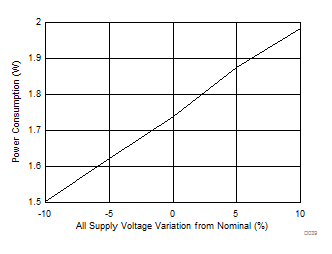DDC bypass mode
Figure 26. Power Consumption vs Supply Voltage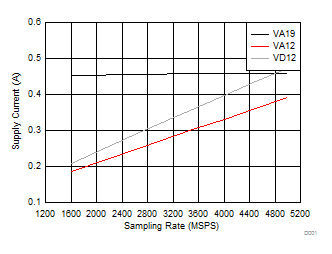DDC bypass mode FIN = 608 MHz
Figure 28. Supply current vs Sampling Rate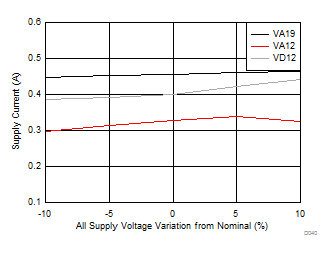DDC bypass mode Foreground calibration mode
Figure 30. Supply Current vs Supply Voltage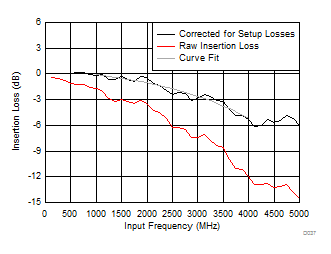Foreground calibration mode
Figure 32. Insertion Loss vs Input Frequency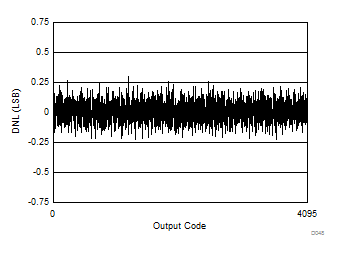Figure 34. DNL versus Code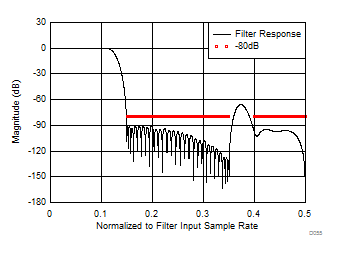Figure 36. Decimate by 4 - Stopband Response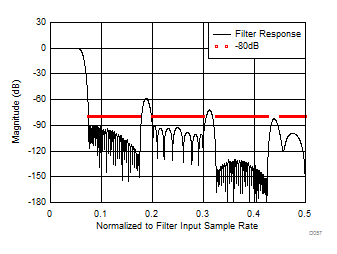Figure 38. Decimate by 8 - Stopband Response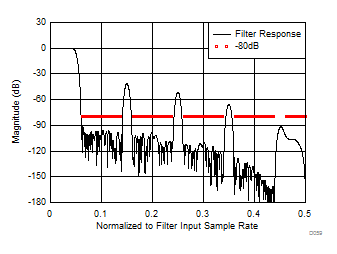Figure 40. Decimate by 10 - Stopband Response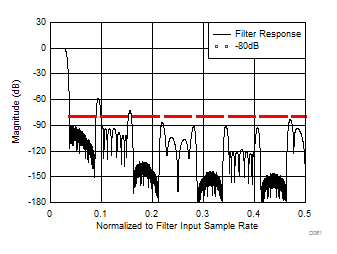Figure 42. Decimate by 16 - Stopband Response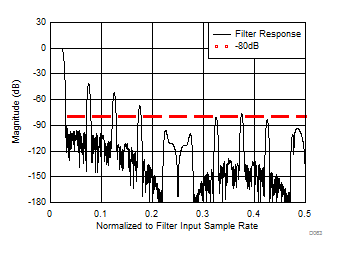Figure 44. Decimate by 20 - Stopband ResponseFigure 46. Decimate by 32 - Stopband Response